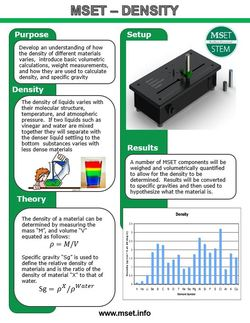# Density

## TECHNICAL OBJECTIVE

The objective of this experiment is to develop an understanding of how the density of different materials varies, introduce basic volumetric calculations, weight measurements and how they are used to calculate density, specific gravity.

## MATERIALS NEEDEDA. Masses

B. Disk

C. 5 kg Load Cell